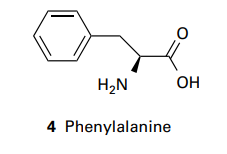×
Get Full Access to Atkins' Physical Chemistry - 11 Edition - Chapter 14b - Problem P14b.5
Get Full Access to Atkins' Physical Chemistry - 11 Edition - Chapter 14b - Problem P14b.5

×ISBN: 9780198769866 2042

## Solution for problem P14B.5 Chapter 14B

Atkins' Physical Chemistry | 11th Edition

• Textbook Solutions
• 2901 Step-by-step solutions solved by professors and subject experts
• Get 24/7 help from StudySoup virtual teaching assistantsAtkins' Physical Chemistry | 11th Edition

4 5 1 295 Reviews
19
1
Problem P14B.5

Phenylalanine (Phe, 4) is a naturally occurring amino acid. What is the energy of interaction between its phenyl group and the electric dipole moment of a neighbouring peptide group? Take the distance between the groups as 0.4nm and treat the phenyl group as a benzene molecule. The dipole moment of the peptide group is $$\mu=2.7 \mathrm{D}$$ and the polarizability volume of benzene is $$\alpha^{\prime}=1.04 \times 10^{-29} \mathrm{~m}^{3}$$.Text Transcription:

mu=2.7 D

alpha^prime=1.04 times 10^-29 m^3

Step-by-Step Solution:
Step 1 of 3

Week 12, Chem 143, Prof. Cracolice Chapter 67: How is Energy Spread out Within Molecules 4/11/16 67.1 How is Energy Spread out Within Molecules  Translational energy­ molecule moving from location to another within its container, energy of random motion  Rotational energy­ energy from tumbling or rotating  Vibrational energy­ diatomic molecule stores energy by vibrations within the bond, similar to a spring stretching and contracting between 2 nuclei  Electronic energy­ attraction among the electrons and the nuclei and the repulsions among the electrons of the molecule  All four times of energy are quantized­ set levels of energy for that molecule  Translational­ very small gaps between levels, rotational­ slightly more spread out but still small gaps  Vibrational­ very large gaps, electronic­ extremely large gaps (usually at ground level of energy)  Spectroscopy­ study of interaction of electromagnetic radiation with matter  Translational and rotational studies with microwaves, vibrational with inferred, electronic with visible and UV radiation ∆ E=hv  , the change in energy is equal to planks constant multiplied by frequency  Total thermodynamic energy­ sum of all of the 4 energy types  Energy at particulate level  number of molecules in each energies are 1 for each type of energy  How does Molecular Energy Relate To Entropy o Macroscopic level analysis of entropy­ pressure, volu

Step 2 of 3

Step 3 of 3

##### ISBN: 9780198769866

Atkins' Physical Chemistry was written by Aimee Notetaker and is associated to the ISBN: 9780198769866. This textbook survival guide was created for the textbook: Atkins' Physical Chemistry, edition: 11. Since the solution to P14B.5 from 14B chapter was answered, more than 203 students have viewed the full step-by-step answer. This full solution covers the following key subjects: . This expansive textbook survival guide covers 327 chapters, and 1120 solutions. The answer to “?Phenylalanine (Phe, 4) is a naturally occurring amino acid. What is the energy of interaction between its phenyl group and the electric dipole moment of a neighbouring peptide group? Take the distance between the groups as 0.4nm and treat the phenyl group as a benzene molecule. The dipole moment of the peptide group is $$\mu=2.7 \mathrm{D}$$ and the polarizability volume of benzene is $$\alpha^{\prime}=1.04 \times 10^{-29} \mathrm{~m}^{3}$$. Text Transcription:mu=2.7 Dalpha^prime=1.04 times 10^-29 m^3” is broken down into a number of easy to follow steps, and 73 words. The full step-by-step solution to problem: P14B.5 from chapter: 14B was answered by Aimee Notetaker, our top Chemistry solution expert on 04/25/22, 03:45PM.

## Discover and learn what students are asking

Statistics: Informed Decisions Using Data : The Normal Probability Distribution
?Find the z-score such that the area to the right of the z-score is 0.483.

Unlock Textbook Solution